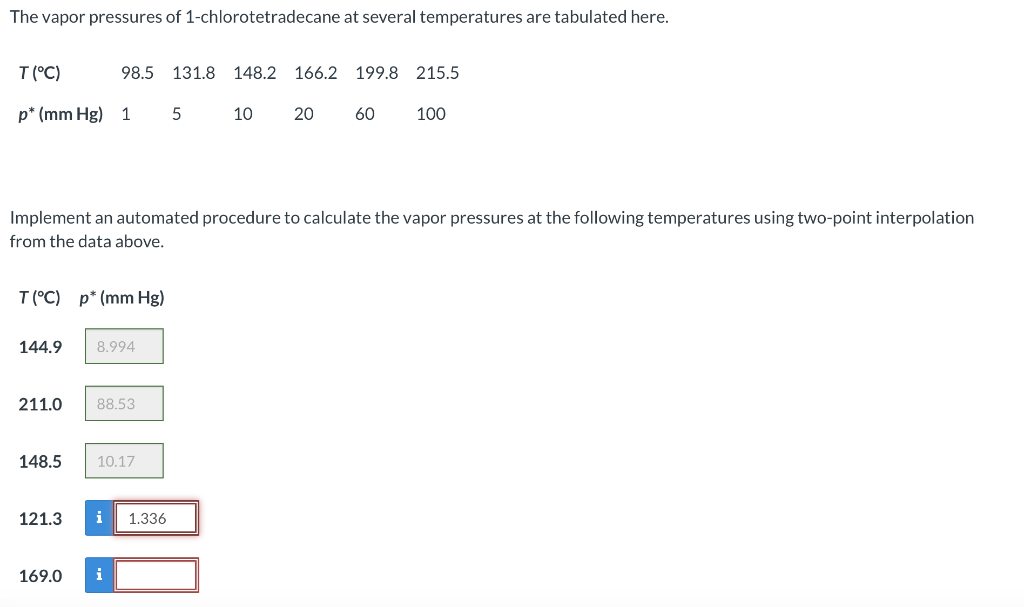# (Solved): The vapor pressures of 1-chlorotetradecane at several temperatures are tabulated here. Implement a ...The vapor pressures of 1-chlorotetradecane at several temperatures are tabulated here. Implement an automated procedure to calculate the vapor pressures at the following temperatures using two-point interpolation from the data above. 144.9 211.0 148.5 121.3 169.0

We have an Answer from Expert

Here we are given the different values of partial pressures at different temperatures. And the intermediate pressure values at intermediate temperature values are calculated using a two-point interpolation method.

Part 1:
Here the partial pressure of 1-chlorotetradecane is to be calculated at,   . Take two points from the tabulated values so that this temperature value lies in between them.

Using the two-point interpolation value, the partial pressure at    is given by:

Substitute the known values, we get

We have an Answer from Expert